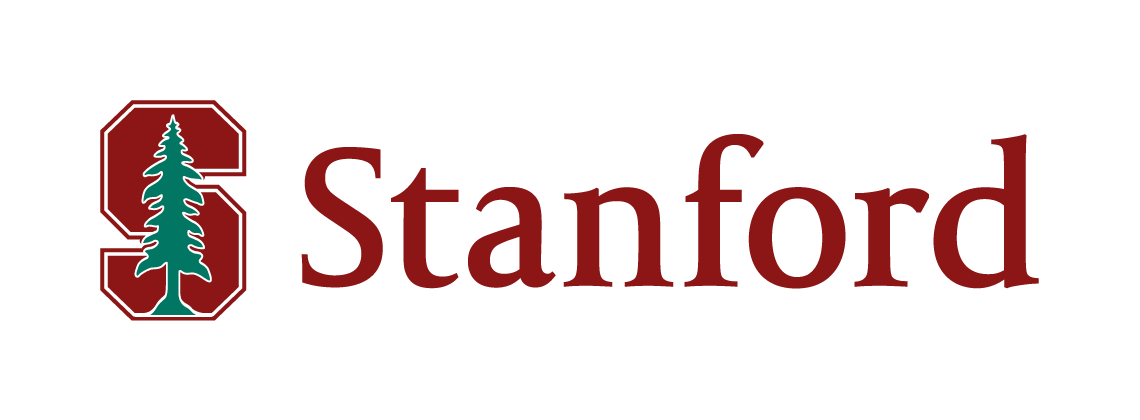## CS254 - Computational Complexity Theory - Fall 2016Instructor: Ryan Williams, Gates 464, 650-723-6690
Office Hours 4:30-5:30pm Wednesdays and Fridays, and by appointment

TA: Huacheng Yu, Gates 488, Office Hours TBA

Time and Place: Wednesdays and Fridays, 3:00-4:20, Building 200, room 305

Join the course discussion on piazza.

### Description

The original theory of computation initiated by Alan Turing (and his contemporaries) studies what is computable in principle, with little regard to physical constraints on computing. In this theory, the only distinguishing characteristic of what is "tractable" to compute (what can be solved) is the difference between the finite and the infinite: algorithms have finite descriptions, "good" algorithms produce answers after finitely many computation steps, "bad" algorithms run for infinitely many steps.

Computational complexity theory studies the impact of limited resources on computation, and gives many new refined answers to the general problem of "what is tractable." For a computational problem we care about solving often, we want to solve it with the minimum necessary resources. What is a resource? Practically anything scarce that a computation could consume: we can measure computation steps, memory usage, computation "size" and "depth" (if we consider Boolean logic circuits), energy consumption, communication between processors, number of processors, number of random bits used, number of qubits used, quality of approximate solutions, number of inputs correct(!) ... the list goes on. (Other names for the field could be "computational resource theory", or "computational measure theory", but "complexity" is darn catchy!) Complexity also studies how we may "trade" one resource for another: if I want to use less memory to solve a problem, how much more time do I need to take?

We study this general problem of limited resources on a completely abstract, mathematical level. It may look strange that it's even possible to mathematically study things like the "amount of time a program takes" when there are gazillions of programming languages, architectures, and operating system issues that could affect running time at any given moment. Nevertheless, we can get a handle on what is efficiently computable at a high level, by starting with Turing's model and carefully defining what resources means, so they will not be model-dependent. This project began in the 1960s, and is now one of the major new mathematical programs in the 21st century. Complexity is, as Arora and Barak put it, an "infant science" -- the best kind of science to get into!

The main goal for this course is to develop this mathematical theory and demonstrate its power for understanding efficient computation. Along the way, we will learn whatever necessary math is needed to get the job done -- complexity theory often uses interesting math in unexpected ways.

• A few problem sets, about two weeks apart.
• A take-home final exam (24 hours -- you can choose the time you take it).
• You may be asked to scribe a lecture in LaTeX.

#### Prerequisites:

This is a graduate course, but is open to anyone. You should probably come with knowledge at the level of CS154 (undergrad automata/complexity), or be ready to pick it up as you go. If you've taken CS161 (undergrad algorithms) that'd probably help too, but it isn't necessary.

#### Textbook:

We will mostly select topics from Part 1 of:
For the first few lectures of the course, Sipser also covers some of the topics. Another reference that is awesome for the intuition (but short on the proofs) is Moore and Mertens' The Nature of Computation. It's like bedtime reading for budding complexity theorists.

### Course Outline

This is a rough plan, subject to change.
1. 9/28    Overview of computational complexity: what we do and why we do it
2. 9/30    Time complexity, $P$ versus $NP$, deterministic time hierarchy theorem
(Arora & Barak, Chapters 2-3)
3. 10/5    $coNP$, nondeterministic time hierarchy theorem, Oracle Turing machines
(A&B, Chapters 2-3), mini-pset out
4. 10/7    (Guest Lecture by Dylan McKay) The polynomial hierarchy: $P^{NP}$, $NP^{NP}$, etc. Start space complexity
(A&B, Chapters 4-5)
5. 10/12    Space complexity: $LOGSPACE$, $PSPACE$, relation to polynomial hierarchy
(A&B, Chapter 4-5) mini-pset due
6. 10/14    $L$ vs $NL$, Savitch's theorem, Boolean circuits
(A&B, Chapter 6) pset 1 out
7. 10/19    Karp-Lipton Theorem, Kannan's Theorem
(A&B, Chapter 6)
8. 10/21    Randomized Algorithms: $BPP$ and Polynomial Identity Testing
(A&B, Chapter 7)
9. 10/26    $BPP$, $BPP \subset P/poly$
(A&B, Chapter 7)
10. 10/28    $BPP \subseteq NP^{NP}$, Counting Complexity: PP, $\#P$
(A&B, Chapter 17) pset 1 due
11. 11/2    The Valiant-Vazirani Theorem
(A&B, Chapter 17) pset 2 out
12. 11/4    THEORY CONFERENCE AT STANFORD!
13. 11/9    Toda's Theorem
(A&B, Chapter 17)
14. 11/11    Interactive Proofs, Deterministic IP = $NP$, Graph Non-Isomorphism
(A&B, Chapter 8)
15. 11/16    Interactive Proofs for $\#SAT$
(A&B, Chapter 8) pset 2 due, pset 3 out
16. 11/18    $IP = PSPACE$
17. 11/23    THANKSGIVING HOLIDAY -- NO CLASS
18. 11/25    THANKSGIVING HOLIDAY -- NO CLASS
19. 11/30    Probabilistically Checkable Proofs
(A&B, Chapter 11 and 22)
20. 12/2    PCPs and Inapproximability
(A&B, Chapter 11 and 22) pset 3 due
21. 12/7    Lower Bounds in Restricted Settings (LOGSPACE vs NP, PARITY vs AC0, NEXP vs ACC)
22. 12/9    Barriers to $P$ vs $NP$: Natural Proofs, Relativization, Algebrization, ..., the Human Brain
(A&B, Chapter 14 and 23)